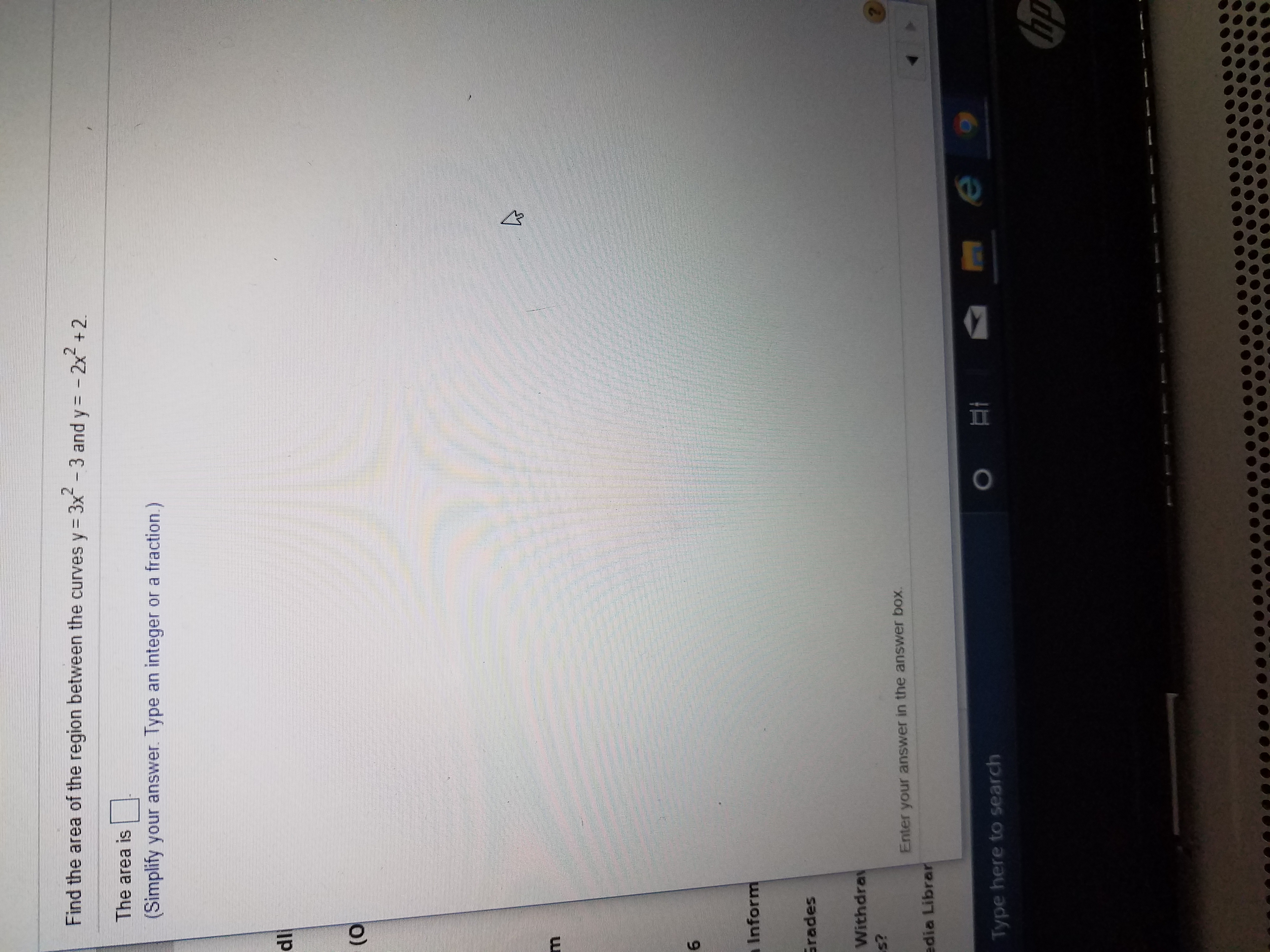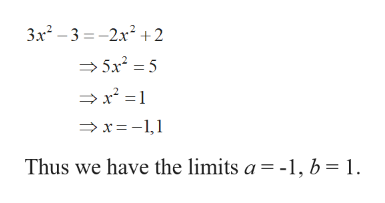# Find the area of the region between the curves y= 3x-3 and y= - 2x + 2.The area is(Simplify your answer. Type an integer or a fraction.)dl(0aInformGradesWithdrays?Enter your answer in the answer box.edia LibrarType here to searchhp

Question
30 viewshelp_outlineImage TranscriptioncloseFind the area of the region between the curves y= 3x-3 and y= - 2x + 2. The area is (Simplify your answer. Type an integer or a fraction.) dl (0 aInform Grades Withdray s? Enter your answer in the answer box. edia Librar Type here to search hp fullscreen
check_circle

Step 1

Given the curves

Step 2

Find the points of intersection by equating the two curves.help_outlineImage Transcriptionclose3x – 3 = -2x² +2 → 5x = 5 >x² = 1 > x= -1,1 Thus we have the limits a = -1, b = 1. fullscreen
Step 3

### Want to see the full answer?

See Solution

#### Want to see this answer and more?

Solutions are written by subject experts who are available 24/7. Questions are typically answered within 1 hour.*

See Solution
*Response times may vary by subject and question.
Tagged in

### Calculus